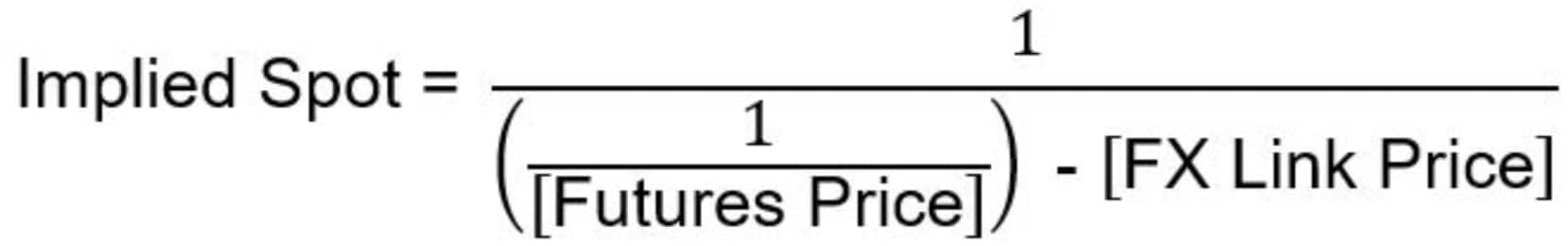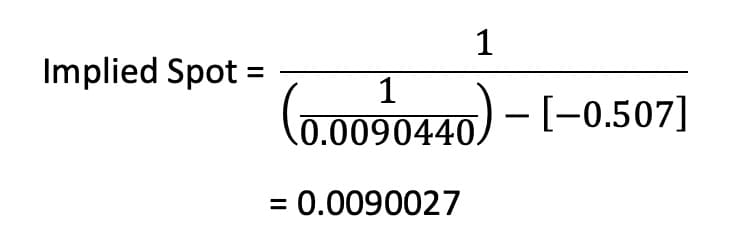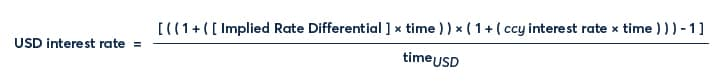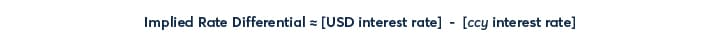# Understanding the CME FX Swap Rate Monitor Methodology

FX Link is a spread between OTC FX spot and FX futures that is traded electronically on the CME central limit order book. At any one time there are spreads available between OTC FX spot and at least the front three futures expiries in eight currency pairs. Trading the spread results in a buy/sell of OTC FX spot versus a sell/buy of FX futures.

As such, FX Link represents the swap points between OTC FX spot and the first 3 monthly listed IMM FX futures, including the front quarterly IMM.

## Overview

• The CME FX Swap Rate Monitor provides analysis of current market pricing within CME Group’s FX Link central limit orderbook.
• The calculator creates the implied interest rate differential for the eight currency pairs supported by FX Link.
• Interest rate differentials shown are “geometric” differentials. At low levels of interest rates in both currencies they are a good estimate of the actual interest rate differentials and can be used in conjunction with known or assumed interest rates in one currency to generate the interest rate in the second currency implied by the FX Link marketplace.
• The interest rate differential implied by the spot and forward FX markets is a useful investment tool.  The theory of covered interest parity argues that forward FX pricing should be a function of the interest rates available in the two currencies, such that the forward FX rate prices in, or compensates for, the interest differential, resulting in investment returns from the two currencies being equivalent.
• In the real world there are other supply and demand factors that affect the forward FX market.  The market implied interest rate differential can be compared to comparative interest rates to highlight potential areas of underperformance or potential investment opportunities.

## Methodology

The CME FX Swap Rate Monitor calculates an implied interest rate differential based on pricing data from CME Group FX futures and the FX Link central limit order book. The value of the differential is the implied rate of interest for US dollars over the rate of interest for the second currency. The value stated is a geometric rate differential as described below.

Each FX futures contract provides an outright FX price for delivery at a fixed date in the future – typically the third Wednesday of the named delivery month. FX Link prices are spread prices between OTC FX spot and the CME Group FX futures. The CME FX Swap Rate Monitor combines the prices from these two orderbooks to calculate the implied interest rate differential.

The first step to calculate the implied interest rate differential is to calculate an outright spot price.  FX Link prices are quoted using the standard OTC convention for the currency pair. Therefore, for EUR, GBP, AUD and NZD, the implied spot price is calculated by subtracting the FX Link price from the futures price. For JPY, CAD, CHF and MXN, where FX Link prices are quoted in USD/ccy terms, the implied spot price is determined as:This results in implied spot prices quoted on a ccy/USD basis for all currency pairs, which is consistent with convention followed by the CME FX futures used in this tool.

The implied interest rate differential is then calculated as:Standard FX market conventions have been used in these calculations.  Rates for EUR, JPY, CHF and MXN are calculated using an Act/360 basis, whilst rates for GBP, AUD, CAD and NZD are calculated using an Act/365 basis.  The number of days in the interest rate period, shown in the monitor as DTE, runs from spot delivery date in the OTC market through to the futures delivery date on the third Wednesday of the month in question.  The spot market convention used is T+2, except for CAD, which is T+1.

The mid-point of the top of book bid and ask prices quoted in both the futures orderbook and the FX Link orderbook are used in these calculations.  The resultant value is therefore an implied mid-rate based on the best available data.

## Examples

### Example 1: EUR/USD

On 27 March 2020, prices in EUR/USD futures and FX Link markets were as follows:

 Bid Ask Mid EUR/USD June 2020 Future 1.10700 1.10705 1.107025 EUR/USD June 2020 FX Link Spread 0.00374 0.00389 0.003815

Using the mid prices, the implied spot price can be determined as 1.107025 – 0.003815 = 1.103210.

There are 78 days between the spot delivery and futures delivery dates.  The implied interest rate differential is therefore calculated as:The interest rate differential implied by the FX Link spread market is 1.5960%.

### Example 2: USD/JPY

On 20 March, prices in JPY/USD futures and FX Link markets were as follows:

 Bid Ask Mid JPY/USD June 2020 Future 0.0090435 0.0090445 0.0090440 USD/JPY June 2020 FX Link Spread -0.522 -0.492 -0.507

Using the mid prices, the implied spot price, in JPY/USD terms, can be determined as:There are 85 days between the spot delivery and futures delivery dates.  The implied interest rate differential is therefore calculated as:The interest rate differential implied by the FX Link spread market is 1.9420%.

## Interpreting the Implied Interest Rate Differential

The value published in the CME FX Swap Rate Monitor is a geometric differential, i.e. it represents the ratio of the interest rates in the two currencies, as follows:Where the implied rate differential and the two interest rates are expressed in percent per annum, and for each rate, time is the number of days from spot delivery to futures delivery divided by the appropriate annualization basis for that currency (e.g. USD has a 360 day annualization basis).

It should be noted that where non-USD interest rates are close to zero, the divisor in the above equation is close to 1, and in this scenario the rate differential can be viewed as a proxy for the implied USD interest rate.  More generally a USD interest rate can be inferred using a known or assumed interest rate in the other currency, i.e.:Similarly, an interest rate for the non-USD currency can be inferred using a known or assumed USD interest rate, i.e.:Whilst the value produced by the CME FX Swap Rate Monitor is a geometric difference, in a low interest rate environment and with the reasonably short terms to maturity that are supported by FX Link, it can be shown that the value is a good approximation for the arithmetic difference between the two interest rates, i.e:As the world’s leading derivatives marketplace, CME Group is where the world comes to manage risk. Comprised of four exchanges - CME, CBOT, NYMEX and COMEX - we offer the widest range of global benchmark products across all major asset classes, helping businesses everywhere mitigate the myriad of risks they face in today's uncertain global economy.

Follow us for global economic and financial news.